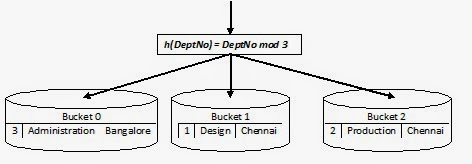Please visit, subscribe and share 10 Minutes Lectures in Computer Science

# Hash Join Technique in DBMS / Simple Hash Join Technique / Sequential Hash Join in Database / Hash join in database example

## Hash Join in database

Hash join is one type of joining techniques that are used to process a join query. Hash join is proposed for performing joins that are Natural joins or Equi-joins. There are several variants of hash joins, like Simple Hash Join, Partitioned Hash Join, and Hybrid Hash Join.

### Simple Hash Join:

Input: The list of tables to be joined, say r and s and the joining attributes r.A and s.B of tables r and s.
Output: Joined relation.

#### Steps:

1. Identify a hash function such that the result of the function should point to one of the identified range of values (or buckets, say bucket 0, bucket 1, …, bucket 9 for example).

2. Identify the smaller relation among the relations that are to be joined, say r and s.

3. Partition the smaller relation, say r, into the identified buckets using the join attribute of r. That is, r is partitioned into available buckets as r0, r1, r2, …, r9. This step is called Building Phase (sometimes Partitioning Phase).

4. Partition the relation s into the identified buckets using the join attribute of s. That is, s is partitioned into available buckets as s0, s1, s2, …, s9. This step is called Probing Phase as the records of s probe for the matching records in the appropriate buckets.

5. Finally the result can be collected from different buckets and submitted as result.

### How does simple Hash join work?

#### Example:

Consider the following two table instances for tables Employee(EmpID, EName, Dept) and Department(DeptNo, DName, DLocation);

 EmpID EName DeptNo E101 Kumar 2 E103 Wess 1 E107 Terry 1 E102 Gowri 2 E110 Morgan 3 E111 Sachin 3
Table 1 - Employee
 DeptNo DName DLocation 1 Design Chennai 2 Production Chennai 3 Administration Bangalore
Table 2 – Department

Step 1: Hash function looks like the one given below;
h(Hash_key) = Hash_key_value mod n
where n is the number of partitions (or buckets). Let us choose the DeptNo attribute as hash key and partition the relation into 3 partitions such as 0, 1, and 2. Then, our hash function will look like as follows;
h(DeptNo) = DeptNo mod 3

Step 2: The Department table is the smaller table.

Step 3: Partition Department using the hash function. The first record of Department will go into Bucket 1, second record into Bucket 2, and third record into Bucket 0.

 DeptNo DName DLocation 1 Design Chennai 2 Production Chennai 3 Administration BangaloreFigure 1 - Hashing of Department table

Step 4: Now partition the larger relation Employee using the same hash function. That is use the following hash function;
h(DeptNo) = DeptNo mod 3
The figure given below shows the partitioning of Employee table into 3 buckets.

 EmpID EName DeptNo E101 Kumar 2 E103 Wess 1 E107 Terry 1 E102 Gowri 2 E110 Morgan 3 E111 Sachin 3Figure 2 - Hashing of Employee table

After successful partitioning, our hash buckets will look the figure given below; in this figure, the first table shows 0th partition of Department table, and the second one shows the 0th partition of Employee table.Figure 3 - Bucket status after hashing of all records of the tables to be joined

Carefully observe the data stored in every bucket. Bucket 0 stores records of zeroth partitions of both tables where the attribute DeptNo (joining attribute) is having the same value, i.e, 3. It is true for other partitions also. Hence, at this stage the join is trivial. This is why this phase of this joining technique is named Probing phase, where the Probe relation searches for the joining attribute value of other relation and gets joined. At this stage, the joining is trivial.

The major advantage is that, if we join with conventional join technique, then the comparison requires,
6 records X 3 records = 18 comparisons
When we use hash join, we need
(1 record X 2 records in Bucket 0)+ (1 X 2 in Bucket 1)+ (1 X 2 in Bucket 2) = 6 comparisons

#### Points to note:

1. Only Equi-join or Natural Join can be performed using Hash join
2. Simple hash join assumed one of the relations as small relation, i.e, the whole relation can fit into memory.
3. Smaller relation is chosen in the building phase.
4. Only a single pass through the tables is required. That is one time scanning of relation is required.
5. Hash function should be chosen such that it should not cause skewness.

## Featured Content

### Multiple choice questions in Natural Language Processing Home

MCQ in Natural Language Processing, Quiz questions with answers in NLP, Top interview questions in NLP with answers Multiple Choice Que...

data recovery